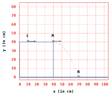# Roll and Drop!

Undeterred247
Homework Statement:
A very small steel marble is shown rolling at a constant speed on a horizontal table. The marble leaves the table at M, falls, and hits the ground at N. This is illustrated in the diagram below which is drawn to scale. Calculate the time it took the marble to travel from L to N.
Relevant Equations:
p(t) = p0 +v0 * t +1/2*a*t^2
a = -g = -9.81m/s^2 = -981cm/s^2
Vertical position (x component):
y(t) = y0 +v0 * t -1/2*g*t^2
Position yx(t) = 0cm = 40cm + 0 *t -1/2 * 981cm/s/s * t^2
edit: I replaced v0 = 0 and got t = 0.286s, which is incorrect according to the submission.

This didn't work so I thought maybe by finding the angle of the throw would help with the following equation but there are still not enough parts to it:
yx(t) = y0 +v0 * sintheta * t -1/2 *g * t^2
yx(t) = 40cm + v0 * sin(36.84) * t -1/2 *981cm/s/s *t^2

Theta = angle of throw where
horizontal = 30cm
vertical = 40cm
hypotenuse = 50cm

#### Attachments

•Screen Shot 2022-05-24 at 11.42.26 AM.png
21.8 KB · Views: 21
Last edited:

Gold Member

That leaves a marble that starts off with v=0 and falls a given distance. You should be able to determine the time of that fall.

Homework Helper
Gold Member
Watch this video. What does it suggest about the time of flight of the marble?

•Lnewqban
Staff Emeritus
Homework Helper
Gold Member
Homework Statement:: A very small steel marble is shown rolling at a constant speed on a horizontal table. The marble leaves the table at M, falls, and hits the ground at N. This is illustrated in the diagram below which is drawn to scale. Calculate the time it took the marble to travel from L to N.
Relevant Equations:: p(t) = p0 +v0 * t +1/2*a*t^2
a = -g = -9.81m/s^2 = -981cm/s^2

Vertical position (x component):
y(t) = y0 +v0 * t -1/2*g*t^2
Position yx(t) = 0cm = 40cm + 0 *t -1/2 * 981cm/s/s * t^2
edit: I replaced v0 = 0 and t = 0.286s, which is incorrect according to the submission.

This didn't work so I thought maybe by finding the angle of the throw would help with the following equation but there are still not enough parts to it:
yx(t) = y0 +v0 * sintheta * t -1/2 *g * t^2
yx(t) = 40cm + v0 * sin(36.84) * t -1/2 *981cm/s/s *t^2

Theta = angle of throw where
horizontal = 30cm
vertical = 40cm
hypotenuse = 50cm

Hello @Undeterred247 .You correctly calculated the time to go from M to N .

You're not finished.

•Undeterred247
Homework Helper
Gold Member
2022 Award
edit: I replaced v0 = 0 and t = 0.286s, which is incorrect according to the submission.
(I assume you meant "I replaced v0 = 0 and got t = 0.286s".)
Maybe you did not read the question correctly. It says "Calculate the time it took the marble to travel from L to N.", not M to N.

Undeterred247
Hello @Undeterred247 .You correctly calculated the time to go from M to N .

You're not finished.
Thank you! How would I use this time from M to N to calculate for the time from L to N?

Homework Helper
Thank you! How would I use this time from M to N to calculate for the time from L to N?
Do you know how far the ball rolls horizontally from L to N?
Do you know how fast it rolls?

Undeterred247
Do you know how far the ball rolls horizontally from L to N?
Do you know how fast it rolls?
I calculated
Vy = Voy +ay *t
Vy = 0 -981 * (0.286)^2
Vy = -80.24

Then took the total distance 60cm/-80.24 = t = -0.748
But when checked this answer is incorrect.

Homework Helper
I calculated
Vy = Voy +ay *t
Vy = 0 -981 * (0.286)^2
Vy = -80.24

Then took the total distance 60cm/-80.24 = t = -0.748
But when checked this answer is incorrect.
Well, yes, that calculation seems to be an incorrect calculation of the wrong thing and does not make any use of the suggestion that was offered.

As nearly as I can determine, it is a garbled calculation of twice the vertical displacement from M to N using cgs units. This is a rather backwards way to proceed since the I believe that the 0.286 s input to the calculation was originally found by using the actual displacement of -40.0 cm as the input.

The factor of two error seems to be from misquoting the SUVAT equation and the 0.24 error is likely from rounding 0.285568... to 0.286. Once that gets fixed, there is the problem of having calculated the wrong thing.

Last edited:
Staff Emeritus
Homework Helper
Gold Member
Thank you! How would I use this time from M to N to calculate for the time from L to N?
Consider the ##x## component of displacement, velocity, and acceleration for both L to M as well as
from M to N .

Undeterred247
Thank you for your help! I figured it out!
The answer is t = 0.572s

•berkeman and Lnewqban